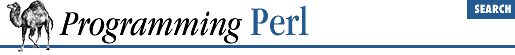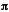home | O'Reilly's CD bookshelfs | FreeBSD | Linux | Cisco | Cisco Exam

#### 3.2.6 atan2

```atan2 ```

Y
```
, ```

X
```
```

This function returns the arctangent of ``` Y ``` ``` /``` ``` X ``` in the range -to. A quick way to get an approximate value ofis to say:

`\$pi = atan2(1,1) * 4;`

For the tangent operation, you may use the ``` POSIX::tan()``` function, or use the familiar relation:

`sub tan { sin(\$_) / cos(\$_) }`